Updating search results...

# 6543 Results

View
Selected filters:
• MathematicsConditional Remix & Share Permitted
CC BY-NC-SA
Rating
0.0 stars

Subject:
Mathematics
Material Type:
Module
Provider:
Georgia Virtual
Author:
Georgia Virtual School
06/02/2018Conditional Remix & Share Permitted
CC BY-NC-SA
Rating
0.0 stars

Middle Grades Math 8 Algebra Part One

Subject:
Mathematics
Material Type:
Module
Provider:
Georgia Virtual
Author:
Georgia Virtual School
06/02/2018Conditional Remix & Share Permitted
CC BY-NC-SA
Rating
2.0 stars

Subject:
Mathematics
Material Type:
Module
Provider:
Georgia Virtual
Author:
Georgia Virtual School
06/02/2018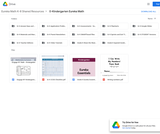Conditional Remix & Share Permitted
CC BY-NC-SA
Rating
5.0 stars

Kindergarten Eureka Math

Subject:
Mathematics
Material Type:
Activity/Lab
Assessment
Author:
Liberty Public Schools
04/07/2021
Remix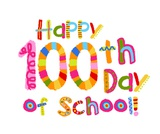Unrestricted Use
CC BY
Rating
0.0 stars

Students will bee able to celebrate the 100th day of school by bringing in a project representing it. They will also fill out a chart that has 100 blank squares for them to fill in.

Subject:
Mathematics
Material Type:
Activity/Lab
Author:
Julie Dameron
11/23/2021Unrestricted Use
CC BY
Rating
0.0 stars

Students will bee able to celebrate the 100th day of school by bringing in a project representing it. They will also fill out a chart that has 100 blank squares for them to fill in.

Subject:
Mathematics
Material Type:
Interactive
Author:
Drew Penn
10/11/2020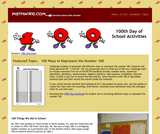Rating
3.26315789474 stars

Resources to mark the 100th day of school with math activities. Challenge students to generate 100 different ways to represent the number 100. Students will easily generate 99 + 1 and 50 + 50, but encourage them to think out of the box. Challenge them to include examples from all of the NCTM Standards strands: number sense, numerical operations, geometry, measurement, algebra, patterns, data analysis, probability, discrete math, Create a class list to record the best entries. Some teachers write 100 in big bubble numeral style and then record the entries inside the numerals.

Subject:
Mathematics
Material Type:
Activity/Lab
Provider:
Mathwire
Author:
Terry Kawas
02/16/2011
Remix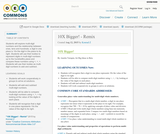Conditional Remix & Share Permitted
CC BY-NC-SA
Rating
0.0 stars

Students will explore multi-digit numbers and the relationship between ones, tens and hundreds; a digit in one place is 10x the digit in the place to its right. Students will use their bodies to represent digits in multi-digit numbers up to the hundredths place and compare these numbers using <, =, >. Students will use their bodies as multi-digit numbers to add and subtract.

Subject:
Mathematics
Material Type:
Activity/Lab
Teaching/Learning Strategy
Author:
08/08/2019
Remix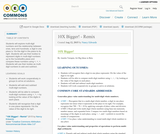Conditional Remix & Share Permitted
CC BY-NC-SA
Rating
0.0 stars

Students will explore multi-digit numbers and the relationship between ones, tens and hundreds; a digit in one place is 10x the digit in the place to its right. Students will use their bodies to represent digits in multi-digit numbers up to the hundredths place and compare these numbers using <, =, >. Students will use their bodies as multi-digit numbers to add and subtract.

Subject:
Mathematics
Material Type:
Activity/Lab
Teaching/Learning Strategy
Author:
Nancy Edwards
08/08/2019
Remix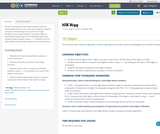Conditional Remix & Share Permitted
CC BY-NC-SA
Rating
0.0 stars

Students will explore multi-digit numbers and the relationship between ones, tens and hundreds; a digit in one place is 10x the digit in the place to its right. Students will use their bodies to represent digits in multi-digit numbers up to the hundredths place and compare these numbers using <, =, >. Students will use their bodies as multi-digit numbers to add and subtract.

Subject:
Mathematics
Material Type:
Activity/Lab
Teaching/Learning Strategy
Author:
Damien Toh
08/08/2019
Remix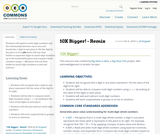Conditional Remix & Share Permitted
CC BY-NC-SA
Rating
0.0 stars

Students will explore multi-digit numbers and the relationship between ones, tens and hundreds; a digit in one place is 10x the digit in the place to its right. Students will use their bodies to represent digits in multi-digit numbers up to the hundredths place and compare these numbers using <, =, >. Students will use their bodies as multi-digit numbers to add and subtract.

Subject:
Mathematics
Material Type:
Activity/Lab
Teaching/Learning Strategy
Author:
JR Dingwall
08/08/2019
Remix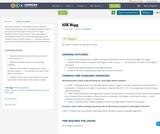Conditional Remix & Share Permitted
CC BY-NC-SA
Rating
0.0 stars

Students will explore multi-digit numbers and the relationship between ones, tens and hundreds; a digit in one place is 10x the digit in the place to its right. Students will use their bodies to represent digits in multi-digit numbers up to the hundredths place and compare these numbers using <, =, >. Students will use their bodies as multi-digit numbers to add and subtract.

Subject:
Mathematics
Material Type:
Activity/Lab
Teaching/Learning Strategy
Author:
Jody Bauer
08/08/2019
Remix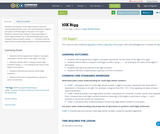Only Sharing Permitted
CC BY-NC-ND
Rating
0.0 stars

Students will explore multi-digit numbers and the relationship between ones, tens and hundreds; a digit in one place is 10x the digit in the place to its right. Students will use their bodies to represent digits in multi-digit numbers up to the hundredths place and compare these numbers using <, =, >. Students will use their bodies as multi-digit numbers to add and subtract.

Subject:
Mathematics
Material Type:
Activity/Lab
Teaching/Learning Strategy
Author:
Sayak Bhattacharyya
08/08/2019
Remix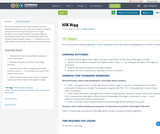Conditional Remix & Share Permitted
CC BY-NC-SA
Rating
0.0 stars

Students will explore multi-digit numbers and the relationship between ones, tens and hundreds; a digit in one place is 10x the digit in the place to its right. Students will use their bodies to represent digits in multi-digit numbers up to the hundredths place and compare these numbers using <, =, >. Students will use their bodies as multi-digit numbers to add and subtract.

Subject:
Mathematics
Material Type:
Activity/Lab
Teaching/Learning Strategy
Author:
Robyn Vsetecka
08/08/2019
RemixConditional Remix & Share Permitted
CC BY-NC-SA
Rating
0.0 stars

Students will explore multi-digit numbers and the relationship between ones, tens and hundreds; a digit in one place is 10x the digit in the place to its right. Students will use their bodies to represent digits in multi-digit numbers up to the hundredths place and compare these numbers using <, =, >. Students will use their bodies as multi-digit numbers to add and subtract.

Subject:
Mathematics
Material Type:
Activity/Lab
Teaching/Learning Strategy
Author:
Keith Mann
08/08/2019
Remix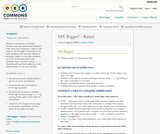Unrestricted Use
CC BY
Rating
5.0 stars

Students will explore multi-digit numbers and the relationship between ones, tens and hundreds; a digit in one place is 10x the digit in the place to its right. Students will use their bodies to represent digits in multi-digit numbers up to the hundredths place and compare these numbers using <, =, >. Students will use their bodies as multi-digit numbers to add and subtract.
Thanks
http://driverrestore.com/

Subject:
Mathematics
Material Type:
Activity/Lab
Lesson Plan
Teaching/Learning Strategy
Author:
Anne Collier
08/08/2019
Remix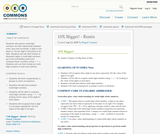Conditional Remix & Share Permitted
CC BY-SA
Rating
0.0 stars

Students will explore multi-digit numbers and the relationship between ones, tens and hundreds; a digit in one place is 10x the digit in the place to its right. Students will use their bodies to represent digits in multi-digit numbers up to the hundredths place and compare these numbers using <, =, >. Students will use their bodies as multi-digit numbers to add and subtract.

Subject:
Mathematics
Material Type:
Activity/Lab
Teaching/Learning Strategy
Author:
Raquel Vazquez
08/08/2019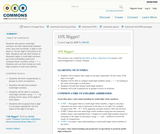Conditional Remix & Share Permitted
CC BY-NC-SA
Rating
3.5 stars

Students will explore multi-digit numbers and the relationship between ones, tens and hundreds; a digit in one place is 10x the digit in the place to its right. Students will use their bodies to represent digits in multi-digit numbers up to the hundredths place and compare these numbers using <, =, >. Students will use their bodies as multi-digit numbers to add and subtract.

Subject:
Mathematics
Material Type:
Activity/Lab
Teaching/Learning Strategy
Author:
01/28/2016
Remix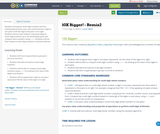Conditional Remix & Share Permitted
CC BY-NC-SA
Rating
0.0 stars

Students will explore multi-digit numbers and the relationship between ones, tens and hundreds; a digit in one place is 10x the digit in the place to its right. Students will use their bodies to represent digits in multi-digit numbers up to the hundredths place and compare these numbers using <, =, >. Students will use their bodies as multi-digit numbers to add and subtract.

Subject:
Mathematics
Material Type:
Activity/Lab
Teaching/Learning Strategy
Author:
Laurie Wyatt
08/08/2019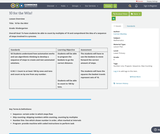Only Sharing Permitted
CC BY-NC-ND
Rating
0.0 stars